# Class 10 RD Sharma Solutions – Chapter 4 Triangles – Exercise 4.5 | Set 1

• Last Updated : 30 Apr, 2021

### Question 1. In fig., ΔACB ∼ ΔAPQ. If BC = 8 cm, PQ = 4 cm, BA = 6.5 cm and AP = 2.8 cm, find CA and AQ.

Solution: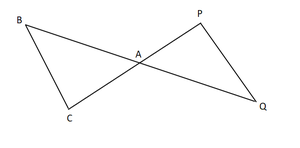Given,

ΔACB ∼ ΔAPQ

BC = 8 cm, PQ = 4 cm, BA = 6.5 cm and AP = 2.8 cm

To find: CA and AQ

We know that,

ΔACB ∼ ΔAPQ             [given]BA/ AQ = CA/ AP = BC/ PQ       [sides are proportional of Similar Triangles]

Now,

6.5/ AQ = 8/ 4

AQ = (6.5 x 4)/ 8

AQ = 3.25 cm

Similarly,

CA/ AP = BC/ PQ

CA/ 2.8 = 8/ 4

CA = 2.8 × 2

CA = 5.6 cm

Hence, CA = 5.6 cm and AQ = 3.25 cm.

### Question 2. In fig., AB ∥ QR, find the length of PB.

Solution: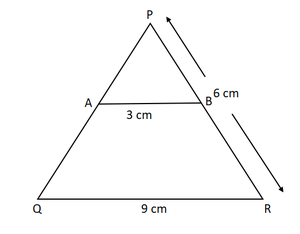Given,

ΔPQR, AB ∥ QR

AB = 3 cm, QR = 9 cm and PR = 6 cm

To find: length of PB

In ΔPAB and ΔPQR

We have,

∠P = ∠P            [Common angle ]

∠PAB = ∠PQR       [Corresponding angles]

∠PBA = ∠PRQ        [Corresponding angles]ΔPAB ∼ ΔPQR          [By AAA similarity criteria]AB/ QR = PB/ PR     [sides are proportional of Similar Triangles]

⇒ 3/ 9 = PB/6

PB = 6/3

Hence the length of PB = 2 cm

### Question 3. In fig. given, XY∥BC. Find the length of XY.

Solution: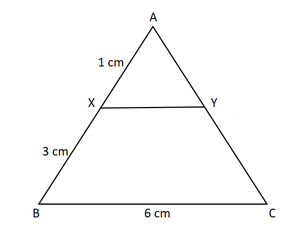Given,

XY∥BC

AX = 1 cm, XB = 3 cm and BC = 6 cm

To find: length of XY

In ΔAXY and ΔABC

We have,

∠A = ∠A             [Common angle]

∠AXY = ∠ABC    [Corresponding angles]

∠AYX = ∠ACB  [Corresponding angles]ΔAXY ∼ ΔABC    [By AAA similarity criteria]XY/ BC = AX/ AB   [Corresponding Parts of Similar Triangles are propositional]

Now,

(AB = AX + XB = 1 + 3 = 4)

XY/6 = 1/4

XY/1 = 6/4

Hence, length of XY = 1.5 cm

### Question 4. In a right-angled triangle with sides a and b and hypotenuse c, the altitude drawn on the hypotenuse is x. Prove that ab = cx.

Solution: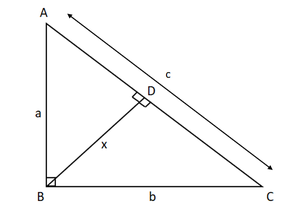Let us consider  ΔABC to be a right angle triangle with sides a,b and c as  hypotenuse. let BD be the altitude drawn on the hypotenuse AC.

To prove: ab = cx

Now,

∠BAC = ∠DAB    [Common]

∠ACB = ∠ABC = 90[right angled triangle]ΔABC ∼ ΔADB              [By AA similarity criteria]

So,

AC/ AB = BC/ EB      [Corresponding Parts of Similar Triangles are propositional]

c/ a = b/ x

⇒ xc = abab = cx

Hence, proved.

### Question 5. In fig., ∠ABC = 90° and BD⊥AC. If BD = 8 cm, and AD = 4 cm, find CD.

Solution: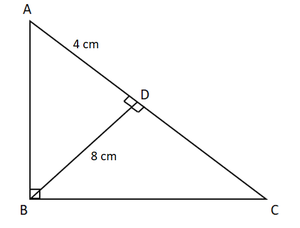Given,

ABC is a right-angled triangle and BD⊥AC.

BD = 8 cm, and AD = 4 cm

To find: CD.

Now in triangle ΔABD and ΔCBD,

∠BDC=∠BDA        [each 90]

∠ABD=∠CBD      [BD⊥AC]ΔDBA∼ΔDCB  [By AA similarity]

(8)2 = 4 x DC

DC = 64/4 = 16 cm

Hence, the length of side CD = 16 cm

### Question 6. In fig., ∠ABC = 90o and BD ⊥ AC. If AB = 5.7 cm, BD = 3.8 cm and CD = 5.4 cm, Find BC.

Solution: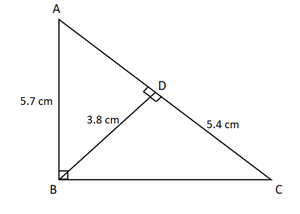Given:

BD ⊥ AC

AB = 5.7 cm, BD = 3.8 cm and CD = 5.4 cm

∠ABC = 90o

Required to find: BC

let ∠BCD=x

∠ADB = ∠CDB = 90o [right angled triangle]

∠ABD = ∠CBD       [∠ABD = ∠CBD = 90o – x]ΔABC ∼ ΔBDC [By AA similarity]

so,

AB/ BD = BC/ CD     [Corresponding Parts of Similar Triangles are propositional]5.7/ 3.8 = BC/ 5.4

BC = (5.7 × 5.4)/ 3.8 = 8.1

Hence, length of side BC = 8.1 cm

### Question 7. In the fig. given, DE ∥ BC such that AE = (1/4)AC. If AB = 6 cm, find AD.

Solution: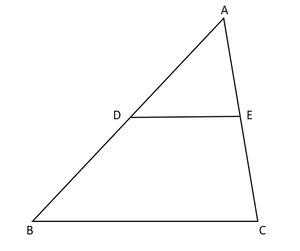Given:

DE∥BC

AE = (1/4)AC

AB = 6 cm.

∠A = ∠A        [Common angle]ΔADE ∼ ΔABC   [By AA similarity criteria]

so,AD/AB = AE/ AC   [Corresponding Parts of Similar Triangles are propositional]AD=1.5 cm

Hence length of AD = 1.5 cm

### Question 8. In the fig. given, if AB ⊥ BC, DC ⊥ BC, and DE ⊥ AC, prove that ΔCED ∼ ΔABC

Solution: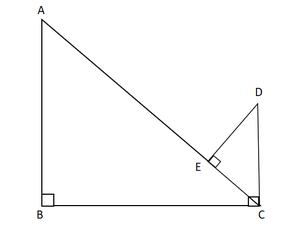Given:

AB ⊥ BC,

DC ⊥ BC,

DE ⊥ AC

To prove: ΔCED∼ΔABC

Now In ΔABC and ΔCED,

∠B = ∠E = 90o   [given]

∠BAC = ∠ECD     [alternate angles]ΔCED∼ΔABC  [AA similarity]

Hence Proved

### Question 9. Diagonals AC and BD of a trapezium ABCD with AB ∥ DC intersect each other at the point O. Using similarity criterion for two triangles, show that OA/ OC = OB/ OD

Solution: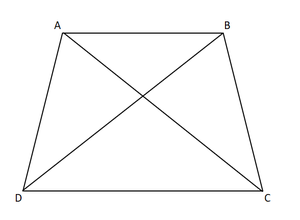Given: OC is the point of intersection of AC and BD in the trapezium ABCD, with AB ∥ DC.

To prove: OA/ OC = OB/ OD

Now In ΔAOB and ΔCOD,

∠AOB = ∠COD [Vertically Opposite Angles]

∠OAB = ∠OCD    [Alternate angles]ΔAOB ∼ ΔCOD

so,OA/ OC = OB/ OD   [Corresponding sides are proportional]

Hence Proved

### Question 10. If Δ ABC and Δ AMP are two right triangles, right angled at B and M, respectively such that ∠MAP = ∠BAC. Prove that

(i) ΔABC ∼ ΔAMP

(ii) CA/ PA = BC/ MP

Solution: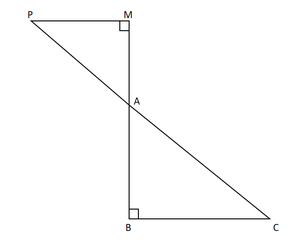(i)  Given:

Δ ABC and Δ AMP are the two right triangles.

Now In ΔABC and ΔAMP,

∠AMP = ∠B = 90o

∠MAP = ∠BAC   [Vertically Opposite Angles]ΔABC∼ΔAMP     [AA similarity]

(ii) Since, ΔABC∼ΔAMP

CA/ PA = BC/ MP  [Corresponding sides are proportional]

Hence, proved.

### Question 11. A vertical stick 10 cm long casts a shadow 8 cm long. At the same time, a tower casts a shadow 30 m long. Determine the height of the tower.

Solution: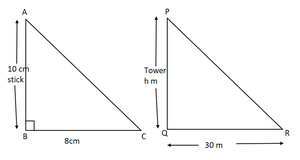Given:

Length of stick = 10cm

Length of the stick’s shadow = 8cm

Length of the tower’s shadow = 30m = 3000cm

To find: the height of the tower = PQ.

Now In ΔABC and ΔPQR,

∠ABC = ∠PQR = 90 [each 90o]

∠ACB = ∠PRQ   [angle are made at the same time]ΔABC ∼ ΔPQR    [By AA similarity]

So,

AB/BC = PQ/QR    [by Corresponding sides are proportional]

10/8 = PQ/ 3000

PQ = (3000×10)/ 8

PQ = 30000/8

PQ = 3750/100

Hence the length of PQ = 37.5 m

### Question 12. In fig., ∠A = ∠CED, prove that ΔCAB ∼ ΔCED. Also, find the value of x.

Solution: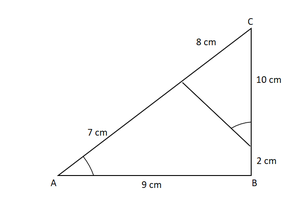Given:

∠A = ∠CED

To prove: ΔCAB ∼ ΔCED

In ΔCAB and ΔCED

∠C = ∠C  [Common]

∠A = ∠CED [Given]ΔCAB ∼ ΔCED [By AA similarity]

so,

CA/ CE = AB/ ED  [by Corresponding sides are proportional]

15/10 = 9/x

x = (9 × 10)/ 15

Hence, value for x = 6 cm

My Personal Notes arrow_drop_up